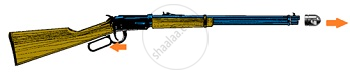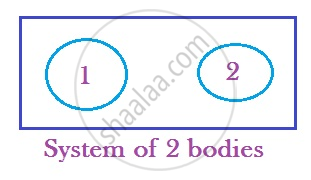# Conservation of Momentum

#### description

• Law of Conservation of Momentum

## Conservation of Momentum

• In an isolated system, the total momentum is conserved.

Example 1. In a Spinning top, total momentum = 0. For every point, there is another point on the opposite side that cancels its momentum.Example 2. Bullet fired from a Rifle
Initially, momentum = 0
Later, the trigger is pulled, bullet gains momentum in àdirection, but this is cancelled by rifle’s ß momentum. Therefore, total momentum = 0During the process, the chemical energy in gunpowder gets converted into heat, sound and chemical energy.

Example 3. Rocket propulsion
Initially, mass of rocket: M. It just started moving with velocity v
Initial momentum = MvLater, gases are ejected continuously in opposite direction with a velocity relative to rocket in downward direction giving a forward push to the rocket.

Mass of the rocket becomes (M-m)
Velocity of the rocket becomes (v + v’)
Final momentum = (M –m) (v + v’)
Thus, Mass × velocity = constant

Collision of BodiesLet the two bodies 1 & 2 have momentum p_1 & p_2 before they collided with each other. After collision their momentum are p_1’ and p_2’ respectively.

By Newton’s Second law,
F = (dp)/(dt)

For 1: F_(12) = (p_1’ – p_1) /(∆t)
For 2: F_(21) = (p_2’ – p_2) /(∆t)

By Newton’s Third law,
F _(12) = - F_(21)
(p_1’ – p_1)/(∆t) = (p_2’ – p_2) /(∆t)
(p_1’ – p_1) = (p_2’ – p_2)
p_1’ + p_2’ = p_1 + p_2

Conclusion:
Final momentum of the system = Initial momentum of the system

Problem: A railway truck A of mass 3 × 104 kg travelling at 0.6 m/s collides with another truck B of half its mass moving in the opposite direction with a velocity of 0.4 m/s. If the trucks couple automatically on collision, find the common velocity with which they move.
Solution.
M1 = 3 × 104 kg
m2 = ½ of mass of A = 1.5 × 104 kg
u1 = 0.6 m/s
u2 = -0.4 m/s

Before collision:
m1u1 + m2u2 = 3 × 104 × 0.6 + 1.5 × 104 ×(-0.4) = 1.2 × 104 kg m/s

After collision:
(m1+ m2) v = 4.5 × 104v kg m/s

As per conservation of momentum,
1.2 × 104 = 4.5 × 104v
V = 1.2/ 4.5 = 0.27 m/s
Therefore, the common velocity is 0.27m/s

If you would like to contribute notes or other learning material, please submit them using the button below.